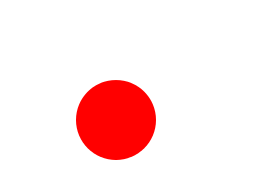# Vector Animations With Python

I am a big fan of Dave Whyte’s vector animations, like this one:It was generated using a special animation language called Processing (here is Dave’s code). While it seems powerful, Processing it is not very elegant in my opinion ; this post shows how to do similar animations using two Python libraries, Gizeh (for the graphics) and MoviePy (for the animations).

## Gizeh and Moviepy

Gizeh is a Python library I wrote on top of cairocffi ( a binding of the popular Cairo library) to make it more intuitive. To make a picture with Gizeh you create a surface, draw on it, and export it:

We obtain this magnificent Japanese flag:To make an animation with MoviePy, you write a function make_frame which, given some time t, returns the video frame at time t:

## Example 1We start with an easy one. In make_frame we just draw a red circle, whose radius depends on the time t:

## Example 2Now there are more circles, and we start to see the interest of making animations programmatically using for loops. The useful function polar2cart transforms polar coordinates (radius, angle) into cartesian coordinates (x,y).

## Example 3Here we fill the circles with a slightly excentred radial gradient to give and impression of volume. The colors, initial positions and centers of rotations of the circles are chosen randomly at the beginning.

## Example 4The shadow is done using a circle with radial fading black gradient whose intensity diminishes when the ball is higher, for more realism (?). The shadow is then squeezed vertically using scale(r,r/2), so that its width is twice its height.

## Example 5This is a derivative of the Dave Whyte animation shown in the introduction. It is made of stacked circles moving towards the picture’s border, with carefully chosen sizes, starting times, and colors (I say carefully chosen because it took me a few dozens random tries). The black around the picture is simply a big circle with no fill and a very very thick black border.

## Example 6You can draw more than circles ! And you can group different elements so that they will move together (here, a letter and a pentagon).

## Example 7We start with just a triangle. By rotating this triangle three time we obtain four triangles which fit nicely into a square. Then we copy this square following a checkerboard pattern. Finally we do the same with another color to fill the missing tiles. Now, if the original triangle is rotated, all the triangles on the picture will also be rotated.

## Example 8A nice thing to do with vector graphics is fractals. We first build a ying-yang, then we use this ying-yang as the dots of a bigger ying-yang, and we use the bigger ying-yang as the dots of an even bigger ying yang etc. In the end we go one level deep into the imbricated ying-yangs, and we start zooming.

## Example 9That one is inspired by this Dave Whyte animation. We draw white-filled circles, each of these being almost completely transparent so that they only add 1 to the value of the pixels that they cover. Pixels with an even value, which are the pixels covered by an even number of circles, are then painted white, while the others will be black. To complexify and have a nicely-looping animation, we draw two circles in each direction, one being a time-shifted version of the other.

## Example 10A pentagon made of rotating squares ! Interestingly, making the squares rotate the other direction creates a very different-looking animation. The squares are placed according to this polar equation.

The difficulty in this animation is that the last square drawn will necessarily be on top of all the others, and not, as it should be, below the first square ! The solution is to draw each frame twice. The first time, we draw the squares starting from the right, so that the faulty square will also be on the right, and we only keep the left part of that picture. The second time we start drawing the squares from the left, so that the faulty square is on the left, and we keep the right part. By assembling the two valid parts we reconstitute a valid picture.

## Mixing videos and vector graphics

A nice advantage of combining Gizeh with MoviePy is that you can read actual video files (or gifs) and use the frames to fill shapes drawn with Gizeh.

We will use this video from the Blender Foundation (it’s under a Creative Common licence). Since you have read until there I’ll show you a little unrelated trick: at 4:32 the rabbit is jumping rope, so there is a potential for a well-looping GIF. We open the video around 4:32, and let MoviePy automatically decide where to cut to have the best-looping GIF possible:Now we can feed the frames of this GIF to Gizeh, using MoviePy’s clip.fl(some_filter), which means “I want a new clip made by transforming the frames of the current clip with some_filter”.Finally, this function adds a zoom on some part of the video.